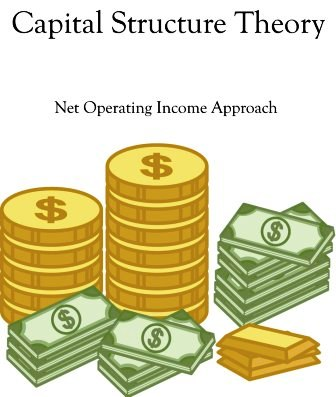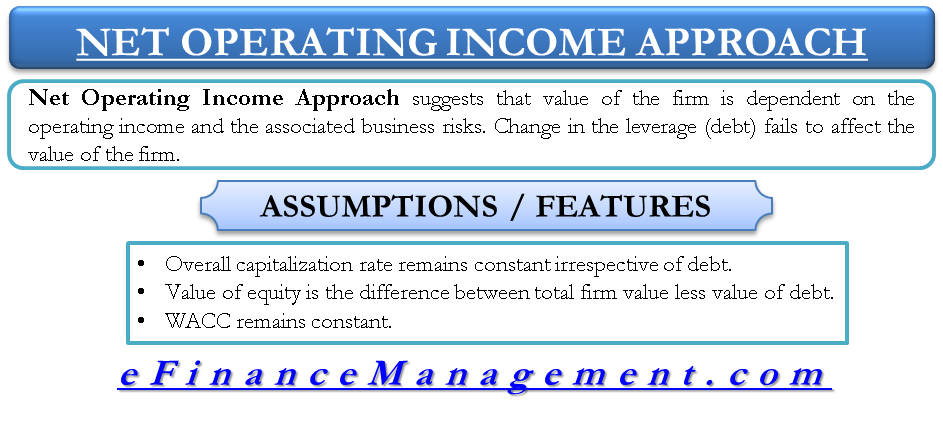# Capital Structure Theory – Net Operating Income Approach

Net Operating Income Approach to capital structure believes that the value of a firm is not affected by the change of debt component in the capital structure. It assumes that the benefit that a firm derives by infusion of debt is negated by the simultaneous increase in the required rate of return by the equity shareholders. With an increase in debt, the risk associated with the firm, mainly bankruptcy risk, also increases and such a risk perception increases the expectations of the equity shareholders.

The capital structure of a company is a mix/ratio of debt and equity in the company’s mode of financing. This ratio of debt in the capital structure is also known as financial leverage. Some companies prefer more of debt while others prefer more of equity while financing their assets. The ultimate goal of a company is to maximize its market value and its profits. In the end, the question stands in front is the relation between the capital structure and value of a firm.There is one school of thought advocating the idea that increasing the debt component or the leverage of a company will increase the value of a firm. On the other hand, increasing the leverage of the company also increases the risk of the company. There are various theories which establish the relationship between financial leverage, weighted average cost of capital and the total value of the firm. One such theory it the Net Operating Income Approach.

## Net Operating Income Approach (NOI Approach)

This approach was put forth by Durand and totally differs from the Net Income Approach. Also famous as traditional approach, Net Operating Income Approach suggests that change in debt of the firm/company or the change in leverage fails to affect the total value of the firm/company. As per this approach, the WACC and the total value of a company are independent of the capital structure decision or financial leverage of a company.

As per this approach, the market value is dependent on the operating income and the associated business risk of the firm. Both these factors cannot be impacted by the financial leverage. Financial leverage can only impact the share of income earned by debt holders and equity holders but cannot impact the operating incomes of the firm. Therefore, change in debt to equity ratio cannot make any change in the value of the firm.

It further says that with the increase in the debt component of a company, the company is faced with higher risk. To compensate that, the equity shareholders expect more returns. Thus, with an increase in financial leverage, the cost of equity increases.## Assumptions / Features of Net Operating Income Approach:

1. The overall capitalization rate remains constant irrespective of the degree of leverage. At a given level of EBIT, the value of the firm would be “EBIT/Overall capitalization rate”
2. Value of equity is the difference between total firm value less value of debt i.e. Value of Equity = Total Value of the Firm – Value of Debt
3. WACC (Weightage Average Cost of Capital) remains constant; and with the increase in debt, the cost of equity increases. An increase in debt in the capital structure results in increased risk for shareholders. As a compensation of investing in the highly leveraged company, the shareholders expect higher return resulting in higher cost of equity capital.

## Example explaining Net Operating Income Approach to Capital Structure:

Consider a fictitious company with below figures. All figures in USD.

 Earnings before Interest Tax (EBIT) = 100,000 Bonds (Debt part) = 300,000 Cost of Bonds issued (Debt) = 10% WACC = 12.5%

Calculating the value of the company:

 (EBIT) = 100,000 WACC = 12.5% Market value of the company = EBIT/WACC = 100,000/12.5% = 800,000 Total Debt = 300,000 Total Equity = Total market value – total debt = 800,000-300,000 = 500,000 Shareholders’ earnings = EBIT-interest on debt = 100,000-10% of 300,000 = 70,000 Cost of equity = 70,000/500,000 = 14%

Now, assume that the proportion of debt increases from 300,000 to 400,000 and everything else remains same.

 (EBIT) = 100,000 WACC = 12.5% Market value of the company = EBIT/WACC = 100,000/12.5% = 800,000 Total Debt = 400,000 Total Equity = Total market value – total debt = 800,000-400,000 = 400,000 Shareholders’ earnings = EBIT-interest on debt = 100,000-10% of 400,000 = 60,000 Cost of equity = 60,000/400,000 = 15%

As observed, in the case of Net Operating Income approach, with the increase in debt proportion, the total market value of the company remains unchanged, but the cost of equity increases.

Last updated on : March 23rd, 2019
1.Ravi kumar chadalapaka
2.faith chisom
3.Talat Mahmud Leeon
4.Oreo
5.Aluf